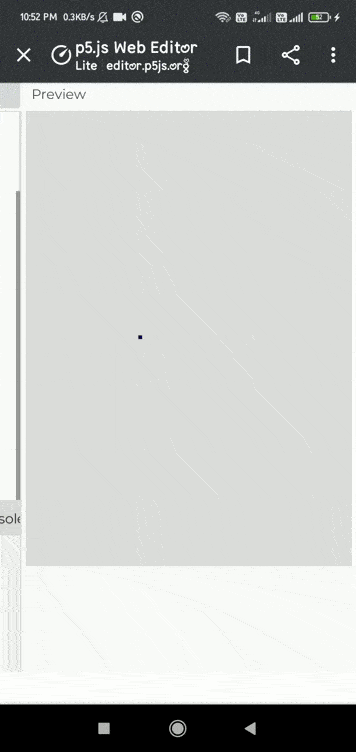# p5.js accelerationZ variable

• Last Updated : 10 May, 2021

The accelerationZ system variable is responsible for the acceleration of the device(tablets or mobile phones) along the Z-axis. It can be used in the draw() function to accelerate the device in the Z-axis of the coordinate.

Its value is represented as meters per second squared.

Syntax:

`accelerationZ`

Example 1:

## Javascript

 `// Move a touchscreen device to register ` `// acceleration changes. ` `function` `draw() { ` `  ``background(220); ` `  ``fill(``'blue'``); ` `   `  `  ``//Set the variable. ` `  ``square(width, height, accelerationZ); ` `}`

Output:Example 2:

## Javascript

 `// Move a touchscreen device to register ` `// Acceleration changes. ` `function` `setup() ` `{ ` `  ``createCanvas(400,400) ` `} ` ` `  `function` `draw() { ` `  ``background(0, 50); ` `   `  `  ``fill(``'green'``); ` `  ``textAlign(CENTER,CENTER); ` `  ``textSize(50); ` `   `  `  ``// Convert the acceleration into integer when ` `  ``// device is moved along x axis. ` `  ``text(int(accelerationZ),windowWidth/2,windowHeight/2); ` `}`

Output:My Personal Notes arrow_drop_up
Recommended Articles
Page :